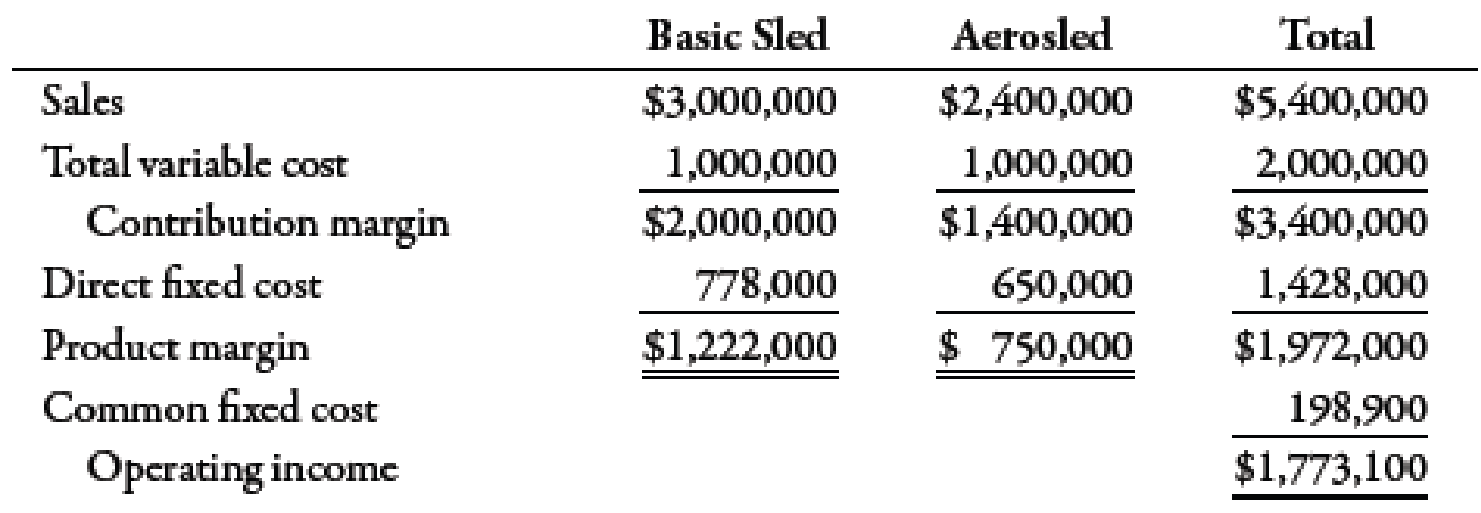Chapter 7, Problem 54P### Managerial Accounting: The Corners...

7th Edition
Maryanne M. Mowen + 2 others
ISBN: 9781337115773

#### Solutions

Chapter
Section### Managerial Accounting: The Corners...

7th Edition
Maryanne M. Mowen + 2 others
ISBN: 9781337115773
Textbook Problem
1 views

# Basu Company produces two types of sleds for playing in the snow: basic sled and aerosled. The projected income for the coming year, segmented by product line, follows:The selling prices are $30 for the basic sled and$60 for the aerosled. (Round break-even packages and break-even units to the nearest whole unit.)Required: 1. Compute the number of units of each product that must be sold for Basu to break even. 2. Assume that the marketing manager changes the sales mix of the two products so that the ratio is five basic sleds to three aerosleds. Repeat Requirement 1. 3. CONCEPTUAL CONNECTION Refer to the original data. Suppose that Basu can increase the sales of aerosleds with increased advertising. The extra advertising would cost an additional $195,000, and some of the potential purchasers of basic sleds would switch to aerosleds. In total, sales of aerosleds would increase by 12,000 units, and sales of basic sleds would decrease by 5,000 units. Would Basu be better off with this strategy? 1. To determine Calculate break-even units for basic sled and aerosled. Explanation Break-Even Point: The point or situation where the amount of total cost is equivalent to total revenue is known as the break-even point. It is the point where there is no loss or no profit. The package of basic sled and aerosled is given below:  Product Price ($) A Unit Variable Cost ($) B Unit Contribution Margin ($) C (A−B) Sales Mix D Package Unit Contribution Margin ($) (C×D) Basic sled 30 103 20 55 100 Aerosled 60 254 35 25 70 Total 170 Table (1) Use the following formula to calculate break-even quantity: Break-even quantity=Total fixed costContribution margin Substitute$1,626,9006 for total fixed cost and $170 for contribution margin in the above formula. Break-even quantity=$1,626,900$170=9,570units Therefore, break-even quantity is 9,570 units. Use the following formula to calculate break-even quantity for basic sled: Break-even quantity=Number of units×Sales mix Substitute 9,570 units for number of units and 55 for sales mix in the above formula. Break-even quantity=9,570×5=47,850units Therefore, the break-even quantity for basic sled is 47,850 units. Use the following formula to compute break-even point for aerosled: Break-even quantity=Number of units×Sales mix Substitute 9,570 units for number of units and 25 for sales mix in the above formula. Break-even quantity=9,570×2=19,140units Therefore, the break-even motorcycle helmet is 19,140 units. Working Note: 1. Calculation of units sold of basic sled: Unitssold=SalesSellingprice=$3,000,000$30=100,000units 2. Calculation of units sold of aerosled: Unitssold=SalesSellingprice=$2,400,000\$60=40,000units

3

2.

To determine

Calculate the break-even units for basic sled and aerosled if the sales mix is 5:3.

3.

To determine

State whether the strategy is profitable.

### Still sussing out bartleby?

Check out a sample textbook solution.

See a sample solution

#### The Solution to Your Study Problems

Bartleby provides explanations to thousands of textbook problems written by our experts, many with advanced degrees!

Get Started